Check the below Online Education NCERT MCQ Questions for Class 6 Maths Chapter 5 Understanding Elementary Shapes with Answers Pdf free download. MCQ Questions for Class 6 Maths with Answers were prepared based on the latest exam pattern. We have provided Understanding Elementary Shapes Class 6 Maths MCQs Questions with Answers to help students understand the concept very well.

Students can also refer to NCERT Solutions for Class 6 Maths Chapter 5 Understanding Elementary Shapes for better exam preparation and score more marks.

## Online Education for Understanding Elementary Shapes Class 6 MCQs Questions with Answers

Understanding Elementary Shapes Class 6 MCQ Question 1.
The angle measure for one complete revolution is
(a) 180°
(b) 360°
(c) 90°
(d) none of these.

Class 6 Maths Chapter 5 MCQ Question 2.
The angle measure for half a revolution is
(a) 90°
(b) 180°
(c) 360°
(d) none of these.

MCQ Questions For Class 6 Maths Chapter 5 Question 3.
The angle measure for one-fourth revolution is
(a) 90°
(b) 360°
(c) 180°
(d) none of these.

Understanding Elementary Shapes Class 6 MCQ With Answers Question 4.
Through what angle measure does the hour hand of a clock turn through, when it goes from 3 to 9?
(a) 90°
(b) 360°
(c) 180°
(d) none of these.

MCQ On Angles For Class 6 Question 5.
Through what angle measure does the hour hand of a clock turn through, when it goes from 5 to 8?
(a) 90°
(b) 180°
(c) 360°
(d) none of these.

MCQ On Understanding Elementary Shapes For Class 6 Question 6.
Through what angle measure does the hour hand of a clock turn through, when it goes from 12 to 9?
(a) 270°
(b) 180°
(c) 360°
(d) 90°.

Understanding Elementary Shapes MCQ Question 7.
Through what angle does the hour hand of a clock turn through, when it goes from 2 to 11?
(a) 270°
(b) 90°
(c) 360°
(d) 180°.

Class 6 Maths Ch 5 MCQ Question 8.
Through what angle does the hour hand of a clock turn through, when it goes from 6 to 3?
(a) 90°
(b) 180°
(c) 270°
(d) 360°.

Ncert Class 6 Maths Chapter 5 MCQ Question 9.
What part of a revolution have you turned through if you stand facing north and turn clockwise to face west?
(a) $$\frac { 1 }{ 4 }$$
(b) $$\frac { 1 }{ 2 }$$
(c) $$\frac { 3 }{ 4 }$$
(d) none of these.

MCQ Class 6 Maths Chapter 5 Question 10.
What part of a revolution have you turned through if you stand facing east and turn clockwise to face west?
(a) $$\frac { 1 }{ 4 }$$
(b) $$\frac { 1 }{ 2 }$$
(c) $$\frac { 3 }{ 4 }$$
(d) none of these.

Class 6 Understanding Elementary Shapes MCQ Question 11.
What part of a revolution have you turned through, if you stand facing north and turn clockwise to face east?
(a) $$\frac { 1 }{ 4 }$$
(b) $$\frac { 1 }{ 2 }$$
(c) $$\frac { 3 }{ 4 }$$
(d) none of these

MCQ On Understanding Elementary Shapes Question 12.
Find the number of right angles turned through by the hour hand of a clock when it goes from 12 to 3.
(a) 1
(b) 2
(c) 3
(d) 4.

Class 6 Math Chapter 5 MCQ Question 13.
Find the number of right angles turned through by the hour hand of a clock when it goes from 4 to 10.
(a) 1
(b) 2
(c) 3
(d) 4.

Class 6 Maths Chapter 5 Extra Questions Question 14.
Find the number of right angles turned through by the hour hand of a clock when it goes from 3 to 12.
(a) 1
(b) 2
(c) 3
(d) 4.

Class 6 Maths Chapter 5 MCQ Questions Question 15.
How many right angles do you make if you start facing north and turn clockwise to south?
(a) 1
(b) 2
(c) 3
(d) 4.

Question 16.
How many right angles do you make if you start facing east and turn clockwise to south?
(a) 1
(b) 2
(c) 3
(d) 4.

Question 17.
How many right angles do you make if you start facing south and turn clockwise to east?
(a) 1
(b) 2
(c) 3
(d) 4.

Question 18.
How many right angles do you make if you start facing east and turn clockwise to east?
(a) 1
(b) 2
(c) 3
(d) 4.

Question 19.
The measure of a right angle is
(a) 45°
(b) 90°
(c) 60°
(d) 180°.

Question 20.
The measure of a straight angle is
(a) 90°
(b) 45°
(c) 180°
(d) 60°.

Question 21.
The measure of an acute angle is
(a) < 90°
(b) > 90°
(c) = 90°
(d) none of these.

Question 22.
The measure of an obtuse angle is
(a) < 90°
(b) > 90° and < 180°
(c) = 90°
(d) none of these.

Question 23.
The measure of a reflex angle is
(a) 180°
(b) < 180°
(c) > 180°
(d) < 90°.

Question 24.
Which of the following angles is the measure of an acute angle?
(a) 30°
(b) 90°
(c) 120°
(d) 210°.

Question 25.
Which of the following angles is the measure of an obtuse angle?
(a) 120°
(b) 90°
(c) 60°
(d) 240°.

Question 26.
Which of the following angles is the measure of a reflex angle?
(a) 90°
(b) 180°
(c) 120°
(d) 270°.

Question 27.
A triangle having three unequal sides is called a
(a) scalene triangle
(b) isosceles triangle
(c) equilateral triangle
(d) right triangle.

Question 28.
A triangle having two equal sides is called
(a) a scalene triangle
(b) an isosceles triangle
(c) an equilateral triangle
(d) a right angled triangle.

Question 29.
A triangle having three equal sides is called
(а) a scalene triangle
(b) an isosceles triangle
(c) an equilateral triangle
(d) a right triangle.

Question 30.
Which of the following statement is true?
(a) The opposite sides of a trapezium are par-allel.
(b) All the sides of a parallelogram are of equal in length.
(c) The diagonals of a square are perpendicular to each other.
(d) All the angles of a rectangle are not equal.

Question 31.
The following shape is of a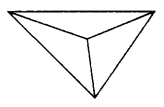(a) cone
(b) cylinder
(c) sphere
(d) pyramid.

Question 32.
The shape is of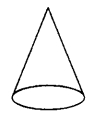(a) cylinder
(b) cone
(c) sphere
(d) cuboid.

Question 33.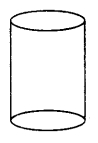The shape is of
(a) cone
(b) cylinder
(c) cuboid
(d) sphere

Question 34.
The shape is of(a) cuboid
(b) cylinder
(c) cone
(d) sphere

Question 35.
The shape is of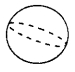(a) cone
(b) cylinder
(c) sphere
(d) Pyramid

Question 36.
The shape is of(a) triangular prism
(b) pyramid
(c) cuboid
(d) cylinder.

Question 37.
The number of faces of the shape is(a) 2
(b) 4
(c) 5
(d) 3

Question 38.
The number of edges of the shape is(a) 4
(b) 8
(c) 10
(d) 12

Question 39.
The number of corners of the shape is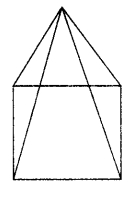(a) 8
(b) 6
(c) 5
(d) 3

Question 40.
The number of faces of the shape is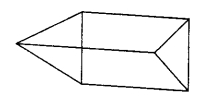(a) 2
(b) 3
(c) 4
(d) 5

Question 41.
The number of edges of the shape is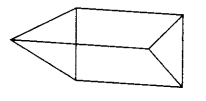(a) 6
(b) 8
(c) 9
(d) 4

Question 42.
The number of comers of the shape is(a) 1
(b) 2
(c) 4
(d) 6

Question 43.
The number of faces of the shape is(a) 1
(b) 2
(c) 3
(d) 4

Question 44.
The number of edges of the shape is(a) 3
(b) 6
(c) 4
(d) 15

Question 45.
The number of vertices of the shape is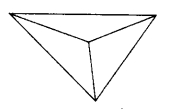(a) 1
(b) 2
(c) 3
(d) 4

Question 46.
The number of faces of the shape is(a) 2
(b) 4
(c) 3
(d) 6

Question 47.
The number of edges of the shape is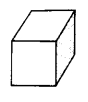(a) 12
(b) 6
(c) 9
(d) 8

Question 48.
The number of vertices of the shape is(a) 4
(b) 6
(c) 5
(d) 8

Question 49.
The number of vertices of a sphere is
(a) 0
(b) 1
(c) 2
(d) none of these.

Question 50.
The number of comers of a cylinder is
(a) 0
(b) 1
(c) 2
(d) none of these.